# RD Sharma Solutions For Class 7 Maths Chapter 2 Fractions Exercise 2.2

The PDF of RD Sharma Solutions for Exercise 2.2 of Class 7 Maths Chapter 2 Fractions are provided here. The questions present in this have been solved by BYJU’S experts in Maths, and this will help students solve the problems without any difficulties. This exercise contains fifteen questions with many sub-questions which deals with the multiplication of integers. By practising sincerely, the students can obtain worthy results in board exams using the RD Sharma Solutions for Class 7.

## Download the PDF of RD Sharma Solutions For Class 7 Maths Chapter 2 – Fractions Exercise 2.2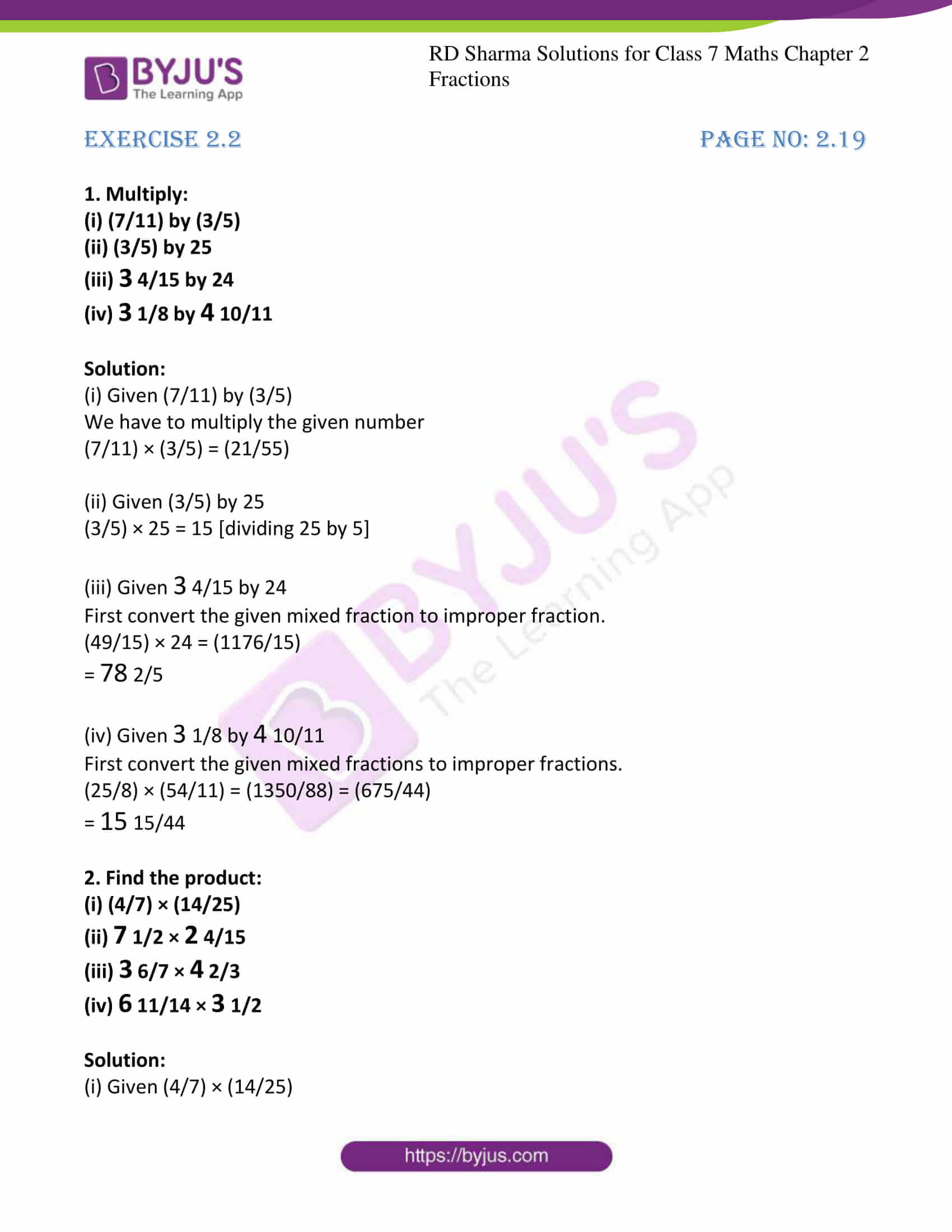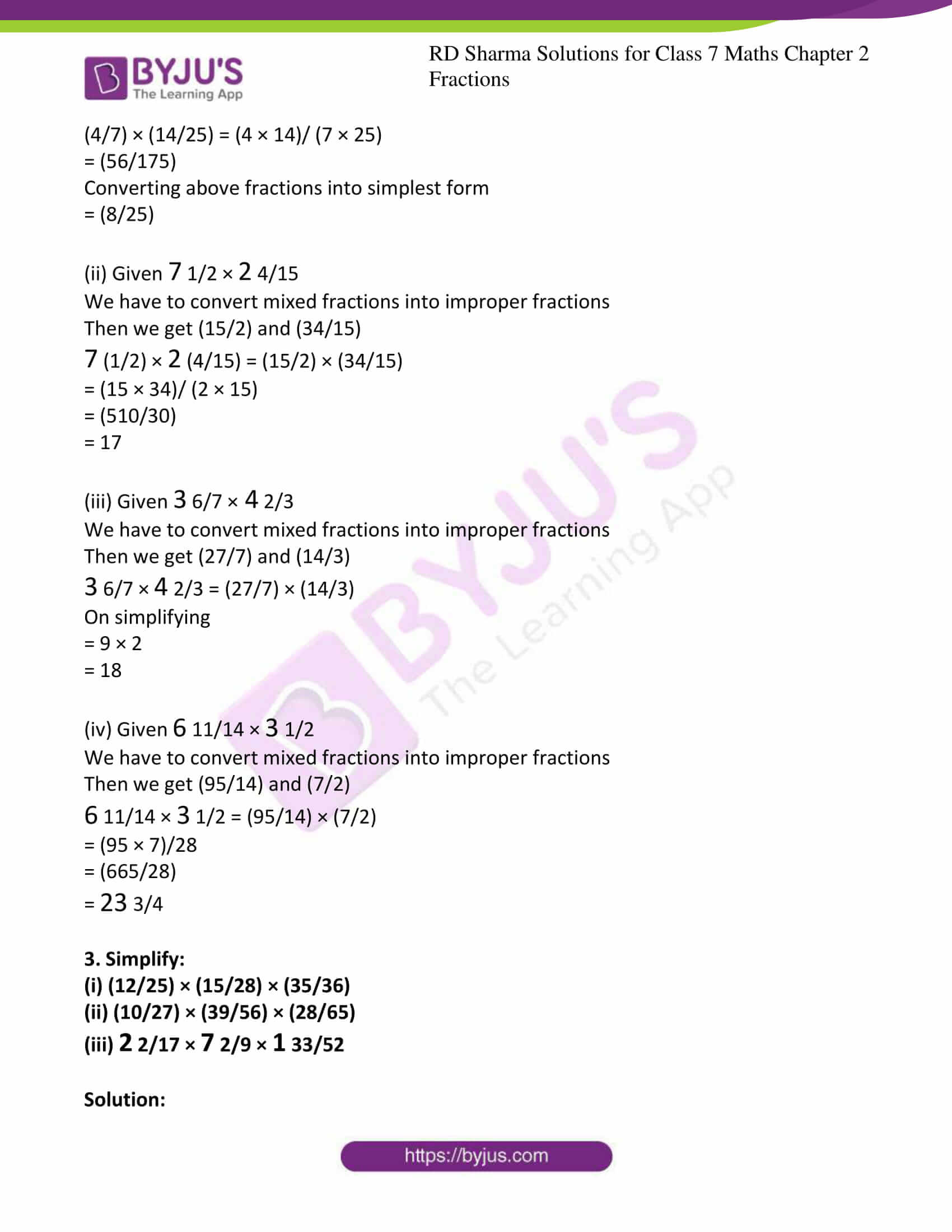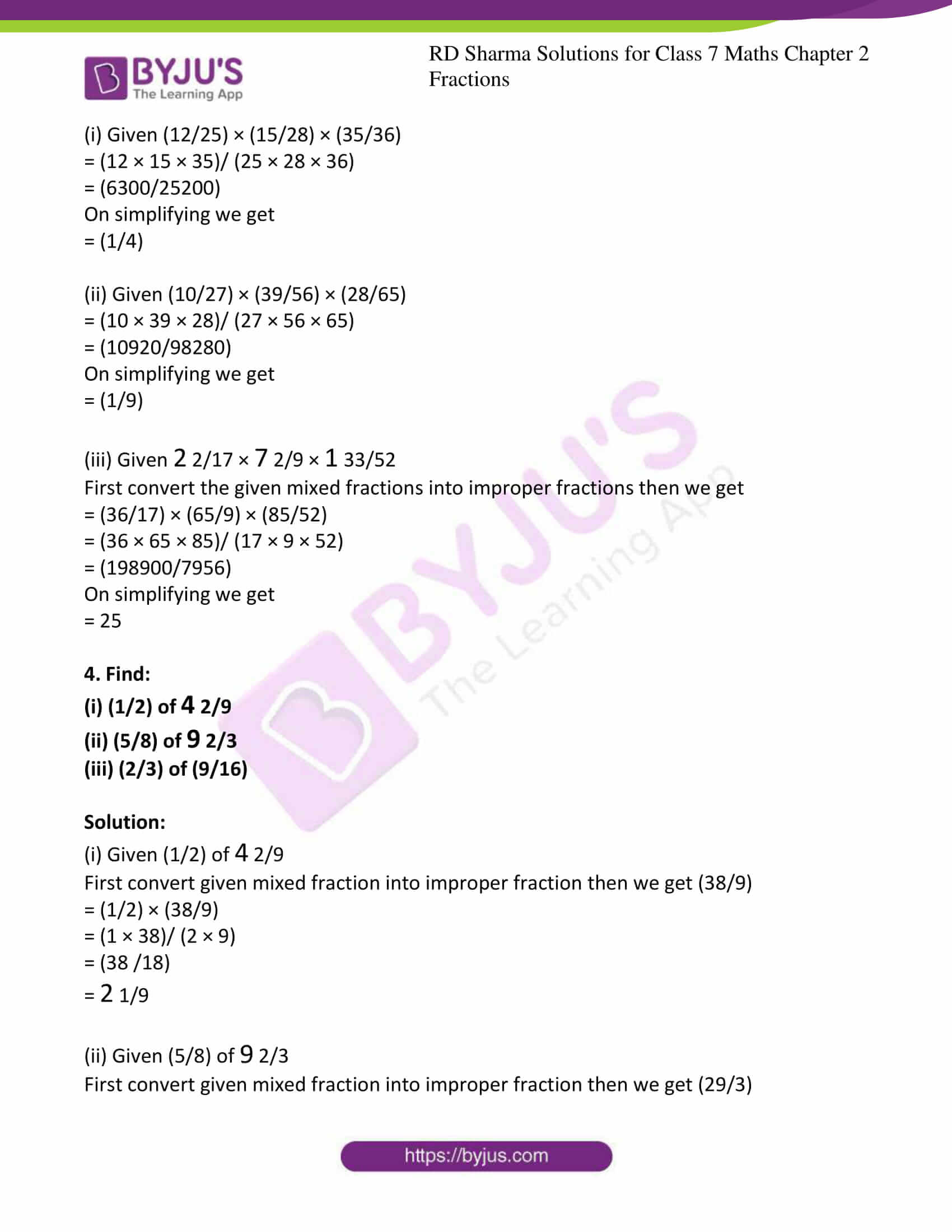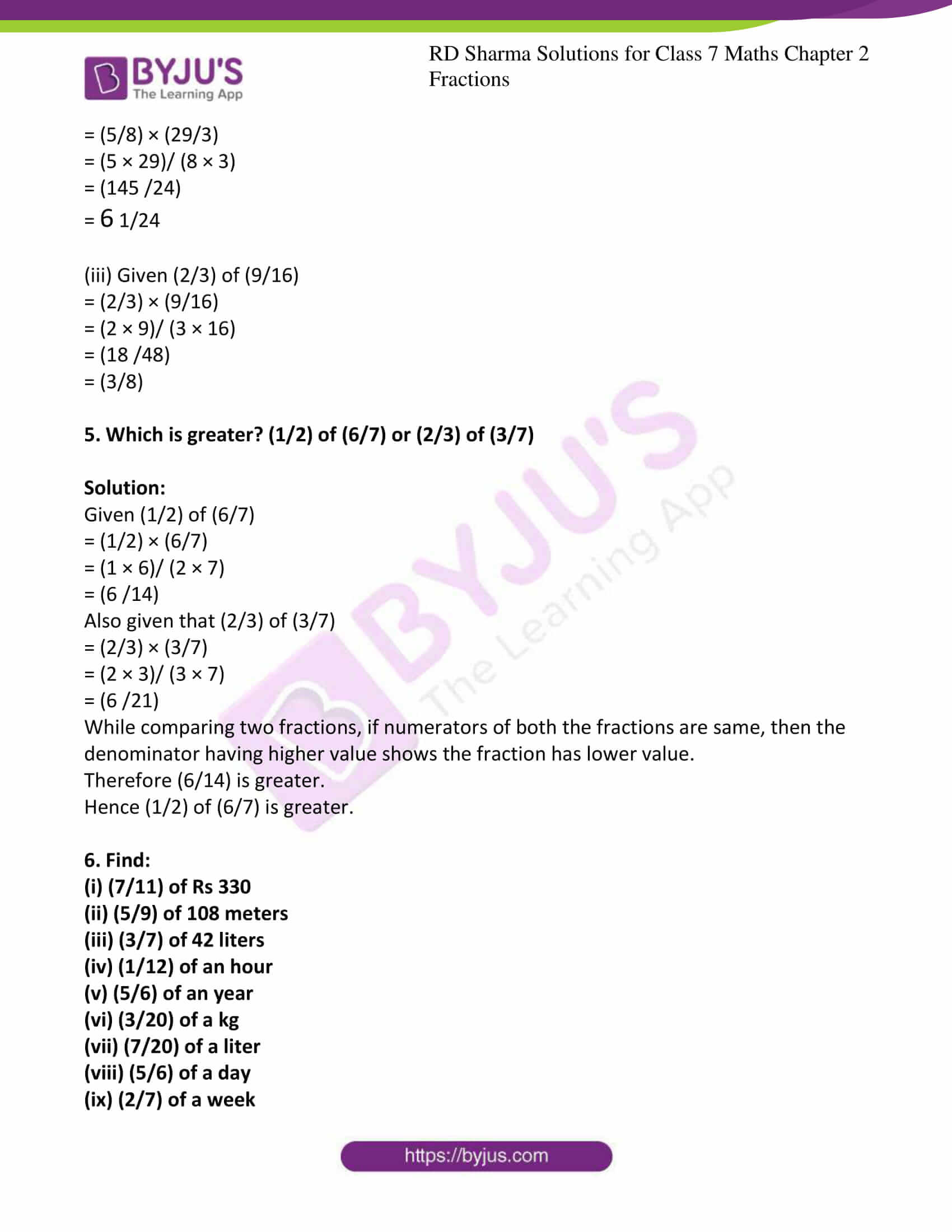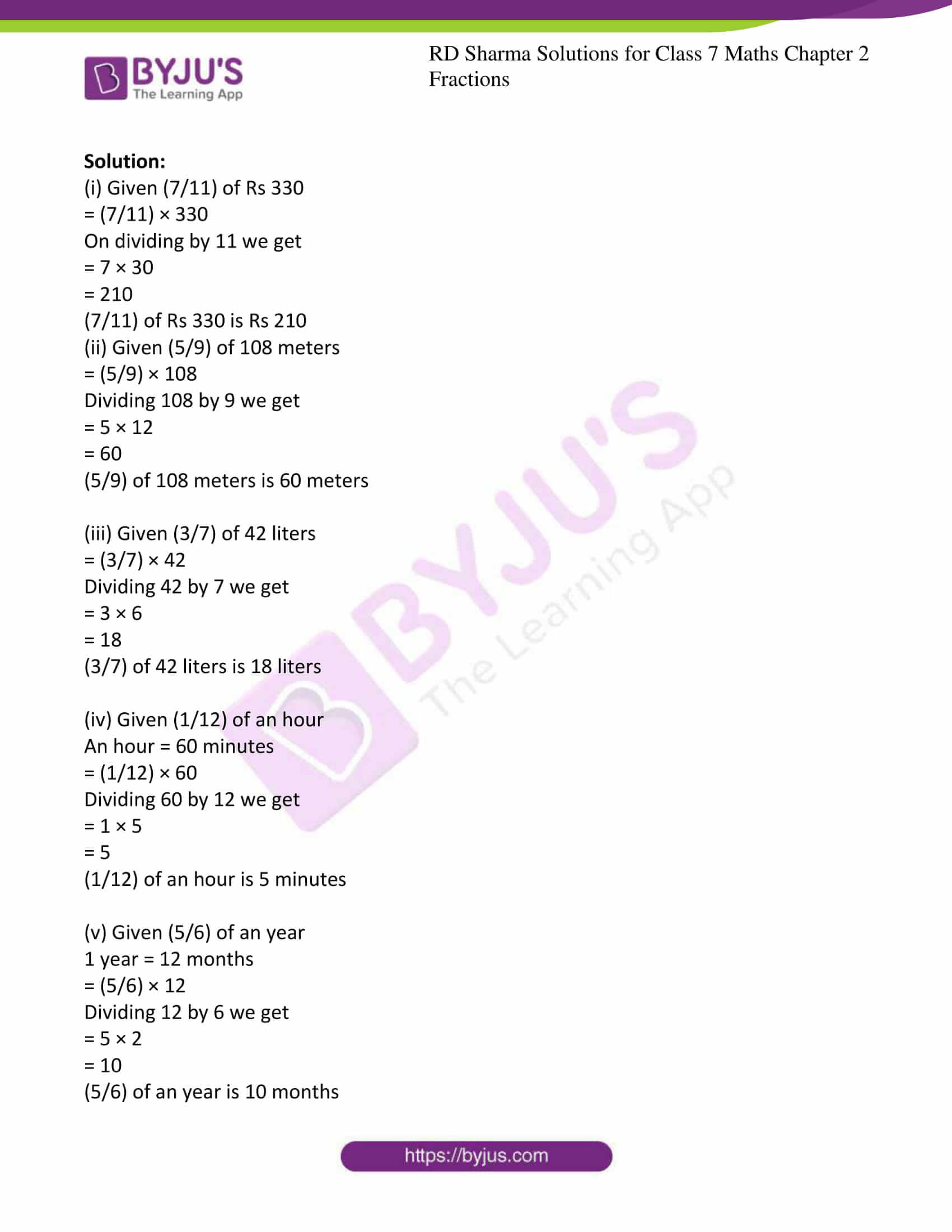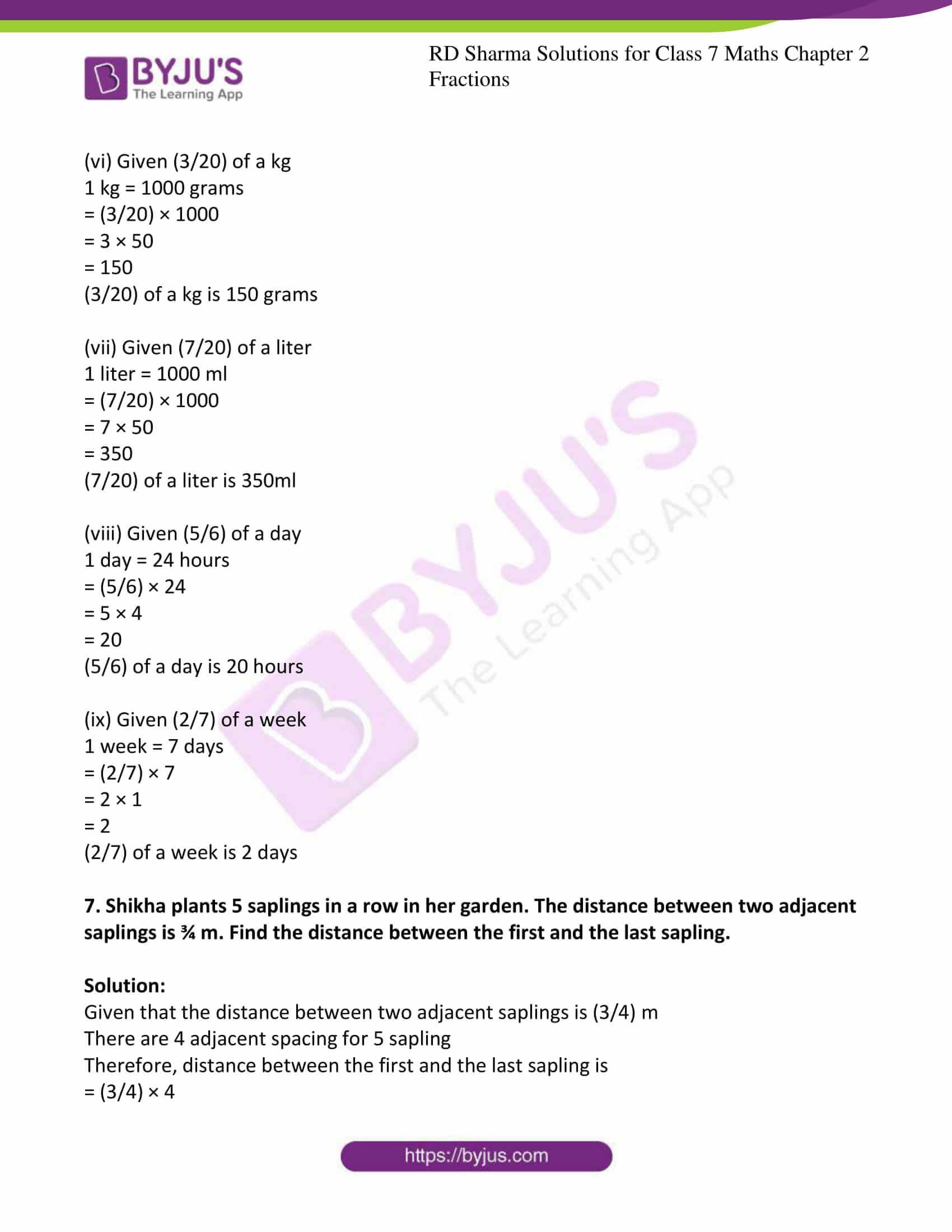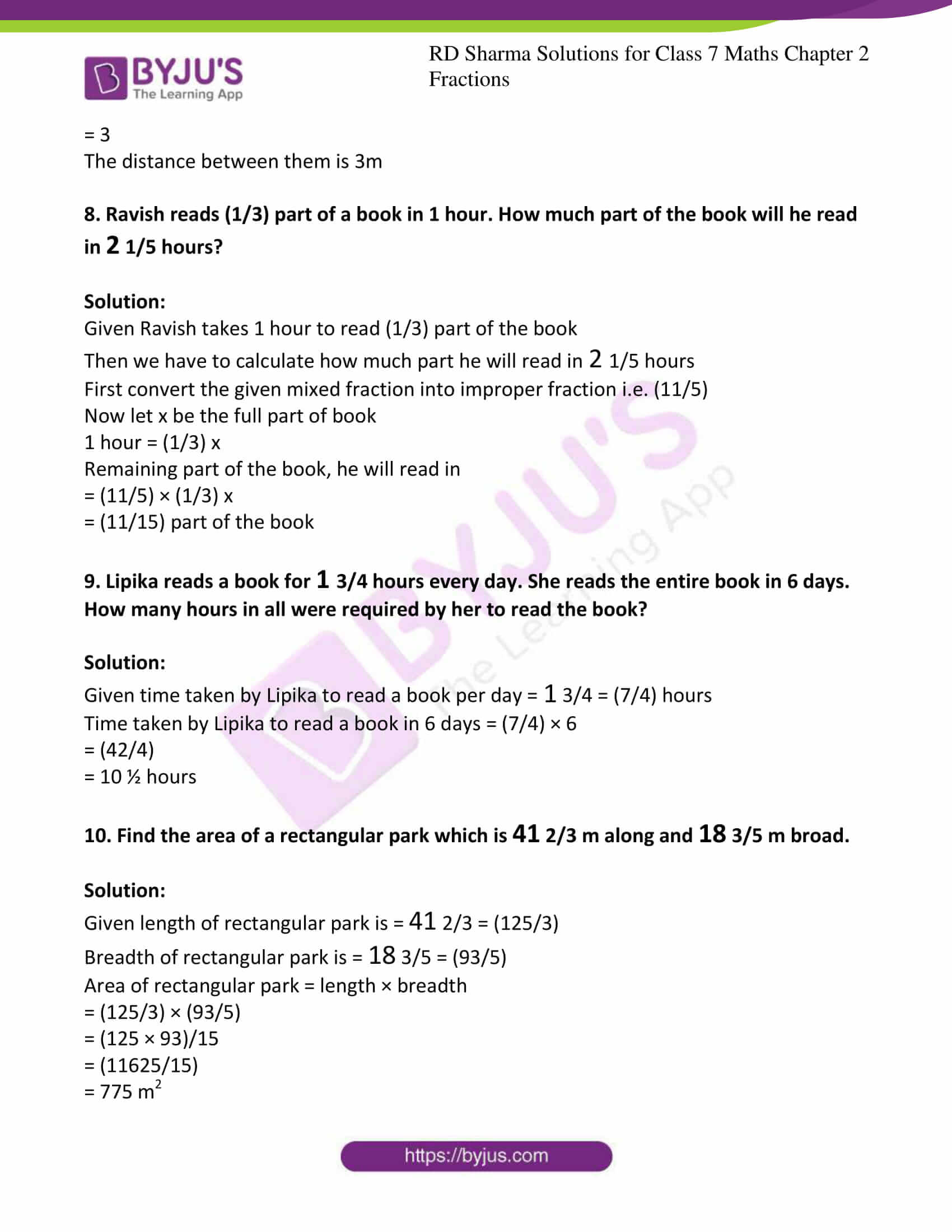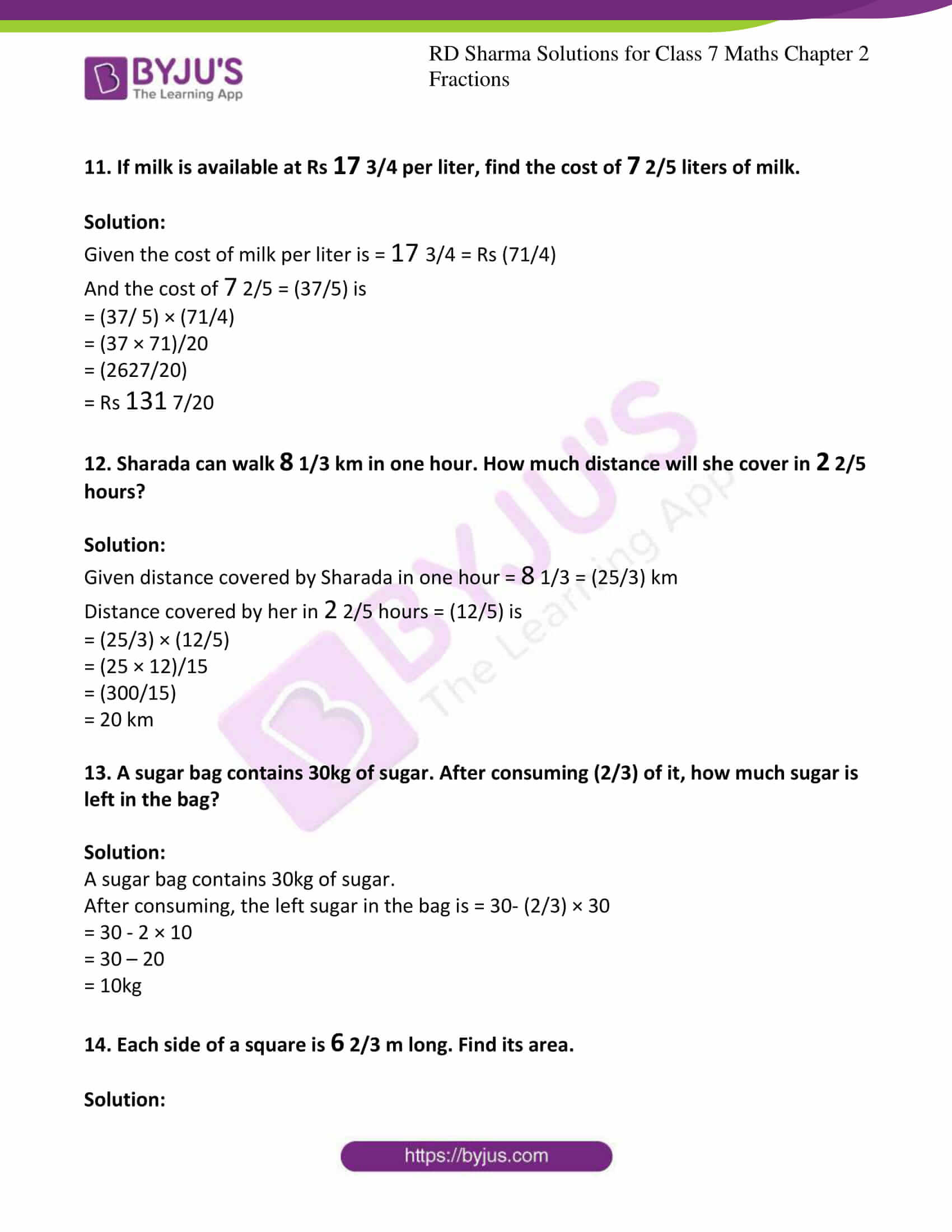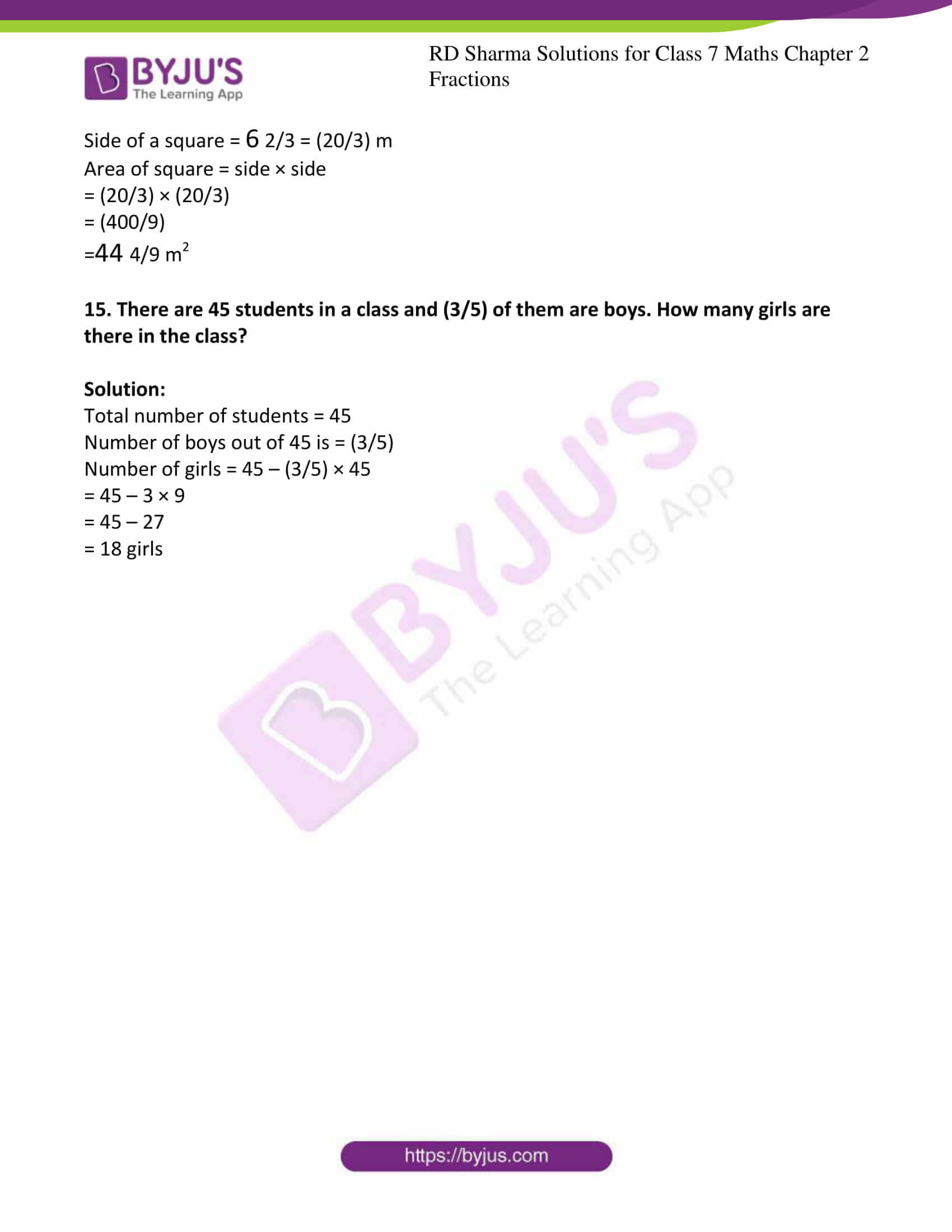### Access answers to Maths RD Sharma Solutions For Class 7 Chapter 2 – Fractions Exercise 2.2

Exercise 2.2 Page No: 2.19

1. Multiply:

(i) (7/11) by (3/5)

(ii) (3/5) by 25

(iii) 3 4/15 by 24

(iv) 3 1/8 by 4 10/11

Solution:

(i) Given (7/11) by (3/5)

We have to multiply the given number

(7/11) × (3/5) = (21/55)

(ii) Given (3/5) by 25

(3/5) × 25 = 15 [dividing 25 by 5]

(iii) Given 3 4/15 by 24

First convert the given mixed fraction to improper fraction.

(49/15) × 24 = (1176/15)

= 78 2/5

(iv) Given 3 1/8 by 4 10/11

First convert the given mixed fractions to improper fractions.

(25/8) × (54/11) = (1350/88) = (675/44)

= 15 15/44

2. Find the product:

(i) (4/7) × (14/25)

(ii) 7 1/2 × 2 4/15

(iii) 3 6/7 × 4 2/3

(iv) 6 11/14 × 3 1/2

Solution:

(i) Given (4/7) × (14/25)

(4/7) × (14/25) = (4 × 14)/ (7 × 25)

= (56/175)

Converting above fractions into simplest form

= (8/25)

(ii) Given 7 1/2 × 2 4/15

We have to convert mixed fractions into improper fractions

Then we get (15/2) and (34/15)

7 (1/2) × 2 (4/15) = (15/2) × (34/15)

= (15 × 34)/ (2 × 15)

= (510/30)

= 17

(iii) Given 3 6/7 × 4 2/3

We have to convert mixed fractions into improper fractions

Then we get (27/7) and (14/3)

3 6/7 × 4 2/3 = (27/7) × (14/3)

On simplifying

= 9 × 2

= 18

(iv) Given 6 11/14 × 3 1/2

We have to convert mixed fractions into improper fractions

Then we get (95/14) and (7/2)

6 11/14 × 3 1/2 = (95/14) × (7/2)

= (95 × 7)/28

= (665/28)

= 23 3/4

3. Simplify:

(i) (12/25) × (15/28) × (35/36)

(ii) (10/27) × (39/56) × (28/65)

(iii) 2 2/17 × 7 2/9 × 1 33/52

Solution:

(i) Given (12/25) × (15/28) × (35/36)

= (12 × 15 × 35)/ (25 × 28 × 36)

= (6300/25200)

On simplifying we get

= (1/4)

(ii) Given (10/27) × (39/56) × (28/65)

= (10 × 39 × 28)/ (27 × 56 × 65)

= (10920/98280)

On simplifying we get

= (1/9)

(iii) Given 2 2/17 × 7 2/9 × 1 33/52

First convert the given mixed fractions into improper fractions then we get

= (36/17) × (65/9) × (85/52)

= (36 × 65 × 85)/ (17 × 9 × 52)

= (198900/7956)

On simplifying we get

= 25

4. Find:

(i) (1/2) of 4 2/9

(ii) (5/8) of 9 2/3

(iii) (2/3) of (9/16)

Solution:

(i) Given (1/2) of 4 2/9

First convert given mixed fraction into improper fraction then we get (38/9)

= (1/2) × (38/9)

= (1 × 38)/ (2 × 9)

= (38 /18)

= 2 1/9

(ii) Given (5/8) of 9 2/3

First convert given mixed fraction into improper fraction then we get (29/3)

= (5/8) × (29/3)

= (5 × 29)/ (8 × 3)

= (145 /24)

= 6 1/24

(iii) Given (2/3) of (9/16)

= (2/3) × (9/16)

= (2 × 9)/ (3 × 16)

= (18 /48)

= (3/8)

5. Which is greater? (1/2) of (6/7) or (2/3) of (3/7)

Solution:

Given (1/2) of (6/7)

= (1/2) × (6/7)

= (1 × 6)/ (2 × 7)

= (6 /14)

Also given that (2/3) of (3/7)

= (2/3) × (3/7)

= (2 × 3)/ (3 × 7)

= (6 /21)

While comparing two fractions, if numerators of both the fractions are same, then the denominator having higher value shows the fraction has lower value.

Therefore (6/14) is greater.

Hence (1/2) of (6/7) is greater.

6. Find:

(i) (7/11) of Rs 330

(ii) (5/9) of 108 meters

(iii) (3/7) of 42 liters

(iv) (1/12) of an hour

(v) (5/6) of an year

(vi) (3/20) of a kg

(vii) (7/20) of a liter

(viii) (5/6) of a day

(ix) (2/7) of a week

Solution:

(i) Given (7/11) of Rs 330

= (7/11) × 330

On dividing by 11 we get

= 7 × 30

= 210

(7/11) of Rs 330 is Rs 210

(ii) Given (5/9) of 108 meters

= (5/9) × 108

Dividing 108 by 9 we get

= 5 × 12

= 60

(5/9) of 108 meters is 60 meters

(iii) Given (3/7) of 42 liters

= (3/7) × 42

Dividing 42 by 7 we get

= 3 × 6

= 18

(3/7) of 42 liters is 18 liters

(iv) Given (1/12) of an hour

An hour = 60 minutes

= (1/12) × 60

Dividing 60 by 12 we get

= 1 × 5

= 5

(1/12) of an hour is 5 minutes

(v) Given (5/6) of an year

1 year = 12 months

= (5/6) × 12

Dividing 12 by 6 we get

= 5 × 2

= 10

(5/6) of an year is 10 months

(vi) Given (3/20) of a kg

1 kg = 1000 grams

= (3/20) × 1000

= 3 × 50

= 150

(3/20) of a kg is 150 grams

(vii) Given (7/20) of a liter

1 liter = 1000 ml

= (7/20) × 1000

= 7 × 50

= 350

(7/20) of a liter is 350ml

(viii) Given (5/6) of a day

1 day = 24 hours

= (5/6) × 24

= 5 × 4

= 20

(5/6) of a day is 20 hours

(ix) Given (2/7) of a week

1 week = 7 days

= (2/7) × 7

= 2 × 1

= 2

(2/7) of a week is 2 days

7. Shikha plants 5 saplings in a row in her garden. The distance between two adjacent saplings is ¾ m. Find the distance between the first and the last sapling.

Solution:

Given that the distance between two adjacent saplings is (3/4) m

There are 4 adjacent spacing for 5 sapling

Therefore, distance between the first and the last sapling is

= (3/4) × 4

= 3

The distance between them is 3m

8. Ravish reads (1/3) part of a book in 1 hour. How much part of the book will he read in 2 1/5 hours?

Solution:

Given Ravish takes 1 hour to read (1/3) part of the book

Then we have to calculate how much part he will read in 2 1/5 hours

First convert the given mixed fraction into improper fraction i.e. (11/5)

Now let x be the full part of book

1 hour = (1/3) x

Remaining part of the book, he will read in

= (11/5) × (1/3) x

= (11/15) part of the book

9. Lipika reads a book for 1 3/4 hours every day. She reads the entire book in 6 days. How many hours in all were required by her to read the book?

Solution:

Given time taken by Lipika to read a book per day = 1 3/4 = (7/4) hours

Time taken by Lipika to read a book in 6 days = (7/4) × 6

= (42/4)

= 10 ½ hours

10. Find the area of a rectangular park which is 41 2/3 m along and 18 3/5 m broad.

Solution:

Given length of rectangular park is = 41 2/3 = (125/3)

Breadth of rectangular park is = 18 3/5 = (93/5)

Area of rectangular park = length × breadth

= (125/3) × (93/5)

= (125 × 93)/15

= (11625/15)

= 775 m2

11. If milk is available at Rs 17 3/4 per liter, find the cost of 7 2/5 liters of milk.

Solution:

Given the cost of milk per liter is = 17 3/4 = Rs (71/4)

And the cost of 7 2/5 = (37/5) is

= (37/ 5) × (71/4)

= (37 × 71)/20

= (2627/20)

= Rs 131 7/20

12. Sharada can walk 8 1/3 km in one hour. How much distance will she cover in 2 2/5 hours?

Solution:

Given distance covered by Sharada in one hour = 8 1/3 = (25/3) km

Distance covered by her in 2 2/5 hours = (12/5) is

= (25/3) × (12/5)

= (25 × 12)/15

= (300/15)

= 20 km

13. A sugar bag contains 30kg of sugar. After consuming (2/3) of it, how much sugar is left in the bag?

Solution:

A sugar bag contains 30kg of sugar.

After consuming, the left sugar in the bag is = 30- (2/3) × 30

= 30 – 2 × 10

= 30 – 20

= 10kg

14. Each side of a square is 6 2/3 m long. Find its area.

Solution:

Side of a square = 6 2/3 = (20/3) m

Area of square = side × side

= (20/3) × (20/3)

= (400/9)

=44 4/9 m2

15. There are 45 students in a class and (3/5) of them are boys. How many girls are there in the class?

Solution:

Total number of students = 45

Number of boys out of 45 is = (3/5)

Number of girls = 45 – (3/5) × 45

= 45 – 3 × 9

= 45 – 27

= 18 girls

## RD Sharma Solutions for Class 7 Maths Chapter – 2 Fractions Exercise 2.2

Exercise 2.2 of RD Sharma Solutions for Class 7 Maths Chapter 2 Fractions explains the fundamental operations on fractions. In this exercise, we shall study on how to multiply mixed fractions with improper fractions, proper fractions and mixed fractions.